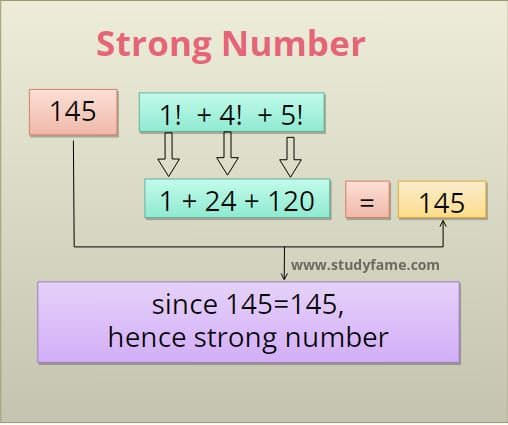# C++ program to check strong number or not

In this example, you will write a C++ program to check whether the user entered integer is a Strong number or not. for example, 1, 2, 145, etc are Strong numbers.

## What is a Strong number?

The Sum of the factorial of individual digits of the number is equal to the original number is called a Strong number.

For example 145 = 1! + 4! + 5! i.e 1 + 24 + 120.## Strong number program in C++

``````
#include<iostream>

using namespace std;
int main() {
int remainders, fact, sum = 0, i, num, copynum;
cout << "enter the number:";
cin >> num;
copynum = num;
while (num != 0)
{
remainders = num % 10;
fact = 1;
/* find the factorial remainders*/
for (i = 1; i <= remainders; i++)
{
fact = fact * i;
}
/* Add factorial of individual digit */
sum = sum + fact;
num = num / 10;
}
if (sum == copynum) {
cout << copynum << " is strong numbers";

} else {
cout << copynum << " is not strong numbers";
}
return 0;
}
``````
output
```enter the number:145
145 is strong numbers```
```enter the number:123
123 is not strong numbers```

## Logic to check Strong number.

1. Take a number from the user and stored it in variable` num`.
2. Iterate the loop until the `num` value becomes 0.
3. Get the last digit of the number and stored it in variable `remainder` i.e remainders = num % 10.
4. find the factorial of the `remainder` stored it in variable `fact`.
5. now add the factorial value to the `sum`.
6. Remove the last digit of a number i.e`num/10`.
7. repeat steps 3 to 6 until num becomes zero.

## C++ program to Check Strong numbers using the function

In this program, you will check Strong Numbers by using User defined function.

``````#include<iostream>
using namespace std;

int checkStrongNumber(int num);
int getfactorial(int remainders);

int main() {
int sum, num;
cout << "enter the number:";
cin >> num;

/* call the function */
sum = checkStrongNumber(num);

if (sum == num) {
cout << num << " is strong numbers";

} else {
cout << num << " is not strong numbers";
}
return 0;
}

int getfactorial(int remainders){
int fact=1,i;
for (i = 1; i <= remainders; i++)
{
fact = fact * i;
}
return fact;
}
int checkStrongNumber(int num){
int remainders, fact, sum = 0;
while (num != 0)
{
remainders = num % 10;
/* find the factorial of remainders using get factorial function*/
fact = getfactorial(remainders);

/* Add factorial of individual digit */
sum = sum + fact;
num = num / 10;
}
return sum;
}
``````

Output of this program will same as the above program